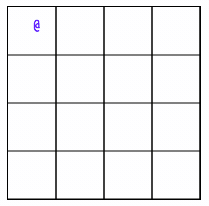## Super Mario Bros. 3 Overworld Map (Part 2)

Last time, we rendered a grid to a canvas element and let the player move an icon around using the arrow keyboard keys.

Next step is going to be to add a transition when moving from one tile to the next. But first, we will need some kind of game loop. Otherwise, we won't get a chance to update the canvas before the animation finishes. The program will be too busy updating the position of the player icon.

``````App.prototype.loop = function () {
this.update()
this.render()

window.requestAnimationFrame(() => {
this.loop()
})
}``````

We can now put our movement logic inside the `update` function and we can be sure that every state change will be rendered to the canvas.

Next, we will move our hero object to it's own class, so that any logic that relates to it can be contained there.

``````class Hero {
constructor (x = 0, y = 0) {
this.position = { x, y }
this.movement = null
this.icon = '@'
}

move () {}

update () {}

render () {}
}``````

The `movement` field will reference an object containing data about a where the player is moving from and to during a given movement. Instead of modifying the player's position, the `move` method will create a `movement` object, which will be used by the `update` method every frame until the movement is over.

``````Hero.prototype.move = function (x, y) {
var startTime = Date.now()
var endTime = startTime + MOVEMENT_DURATION

this.movement = {
vector: { x, y },
start: {
x: this.position.x,
y: this.position.y,
time: startTime
},
end: {
x: this.position.x + x,
y: this.position.y + y,
time: endTime
}
}
}``````

We're keeping track of when the movement started and is scheduled to stop, where it is going from and to, and what is the directional vector.

``````Hero.prototype.update = function () {
if (this.movement) {
let now = Date.now()

if (now < this.movement.end.time) {
let ratio = (now - this.movement.start.time) / MOVEMENT_DURATION

let x = this.movement.start.x + (this.movement.vector.x * ratio)
let y = this.movement.start.y + (this.movement.vector.y * ratio)

this.position.x = x
this.position.y = y
} else {
this.position.x = this.movement.end.x
this.position.y = this.movement.end.y
this.movement = null
}
}
}``````

Every frame, if there is a movement in progress, we want to determine how far we are into the movement, and use that to compute the appropriate position. If the movement has reached it's end time, we want to make sure to set the player to it's predetermined ending position, and get rid of the `movement` object.

We can then move the rendering code for the player into this class as well, to keep our code organized. We pass it a reference to the rendering context since it is not available from within this class.

``````Hero.prototype.render = function (ctx) {
ctx.fillStyle = 'blue'
ctx.fillText(
this.icon,
this.position.x * TILE_SIZE + HALF_TILE,
this.position.y * TILE_SIZE + HALF_TILE
)
}``````

We now have smooth movement from tile to tile.In the next part, we will be looking at rendering tiles and managing collisions.

I don't have a newsletter right now.